# Demystifying the Fourier transform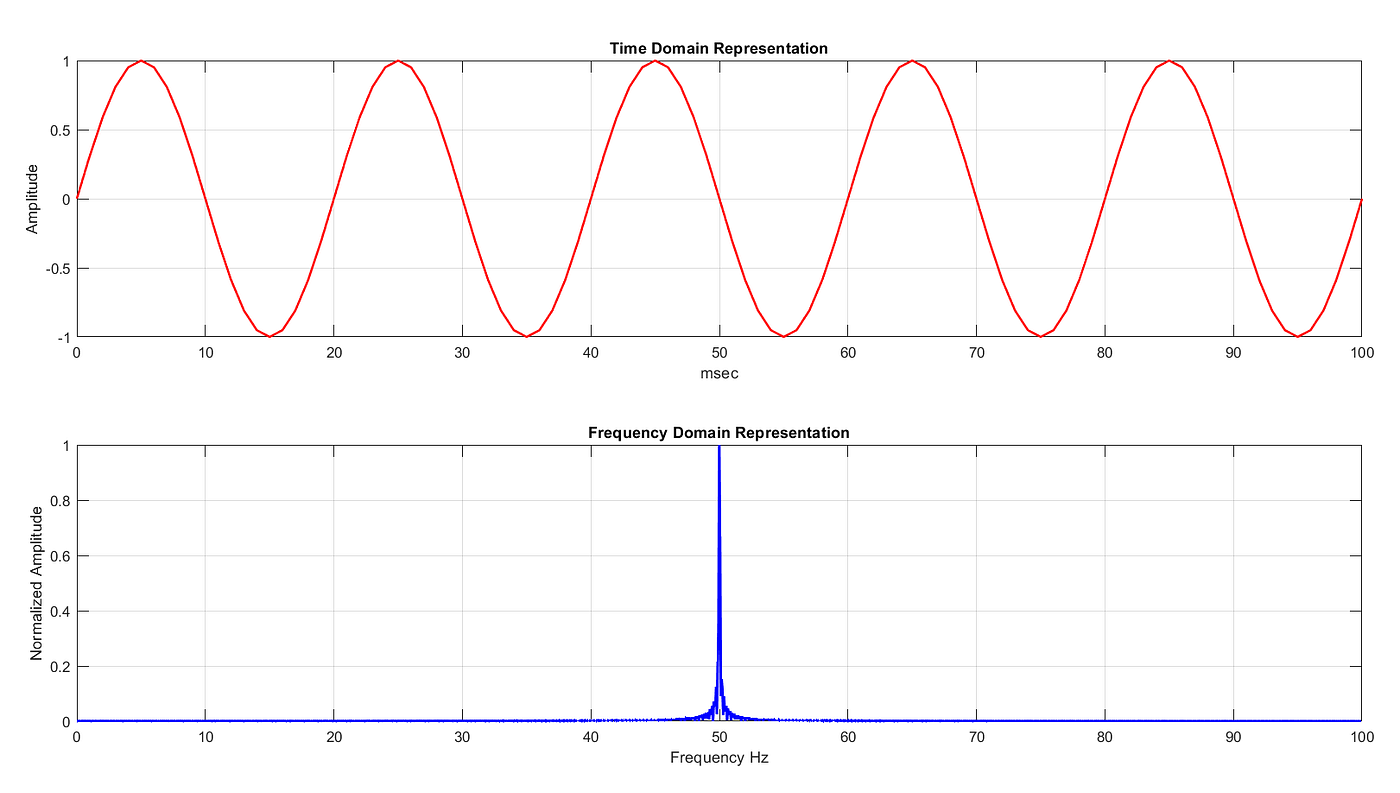Figure 1. Time and frequency domain representation of a signal with a single frequency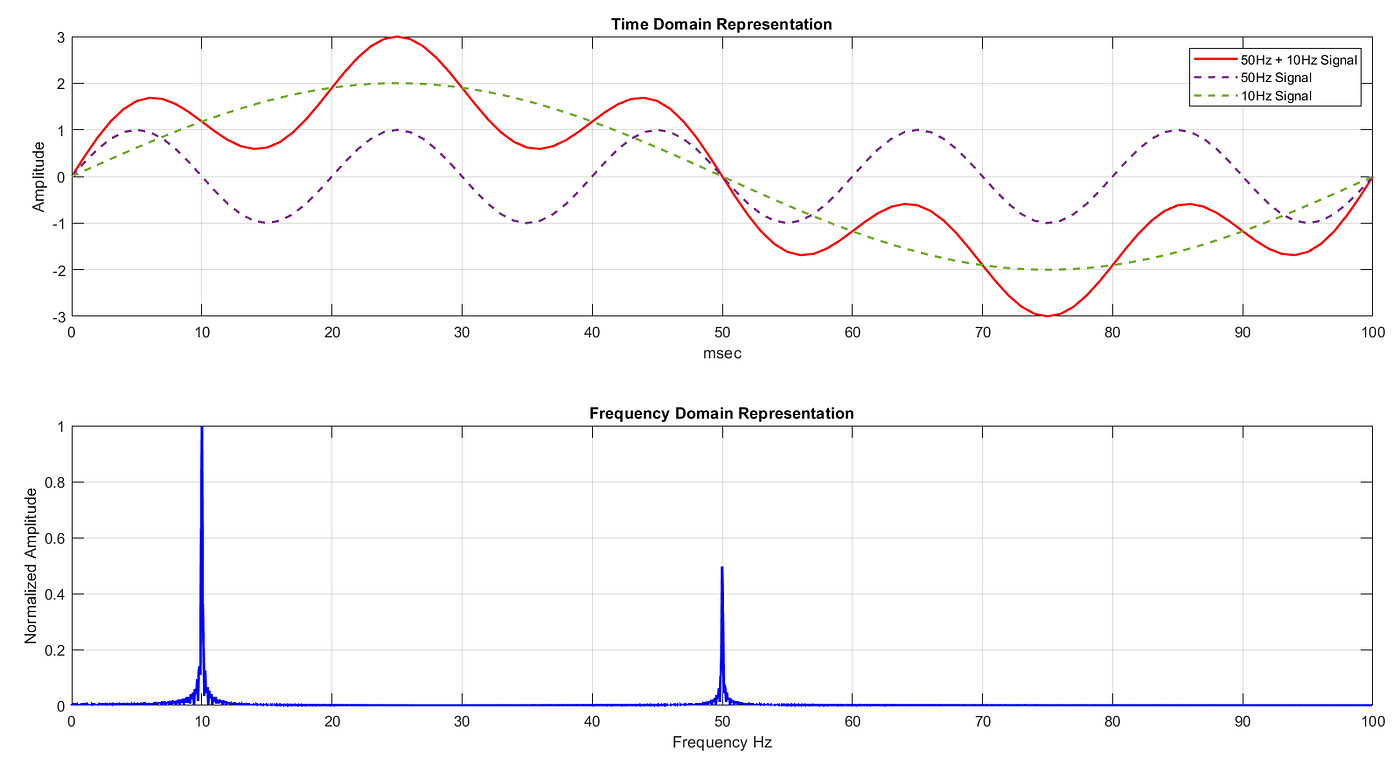Figure 2. Time and frequency domain representation of a signal with multiple frequencies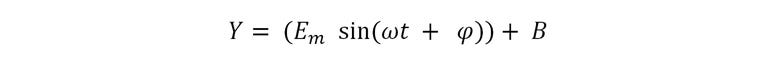Eq1. Equation of a sine wave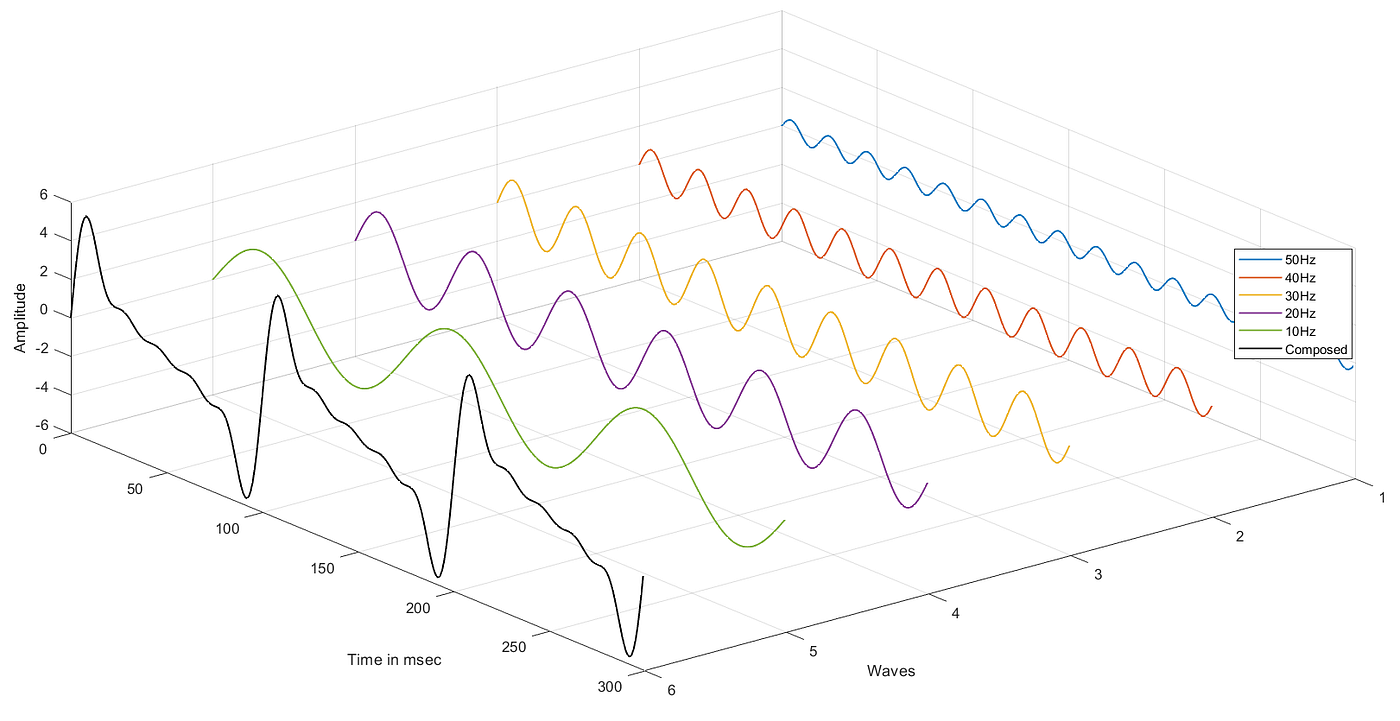Figure 3. Fourier Series Representation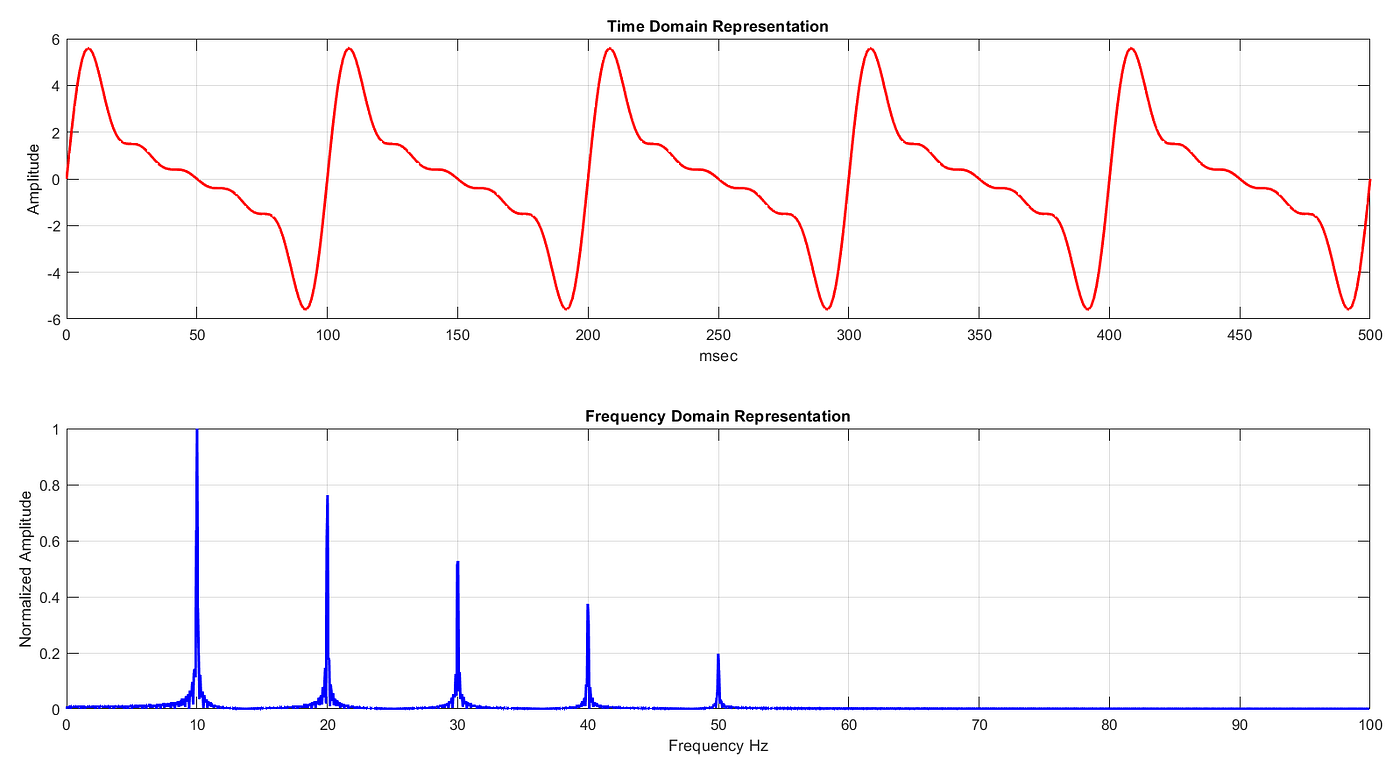Figure 4. Signal Decomposition by Fourier TransformEq2. Equation of Fourier Transform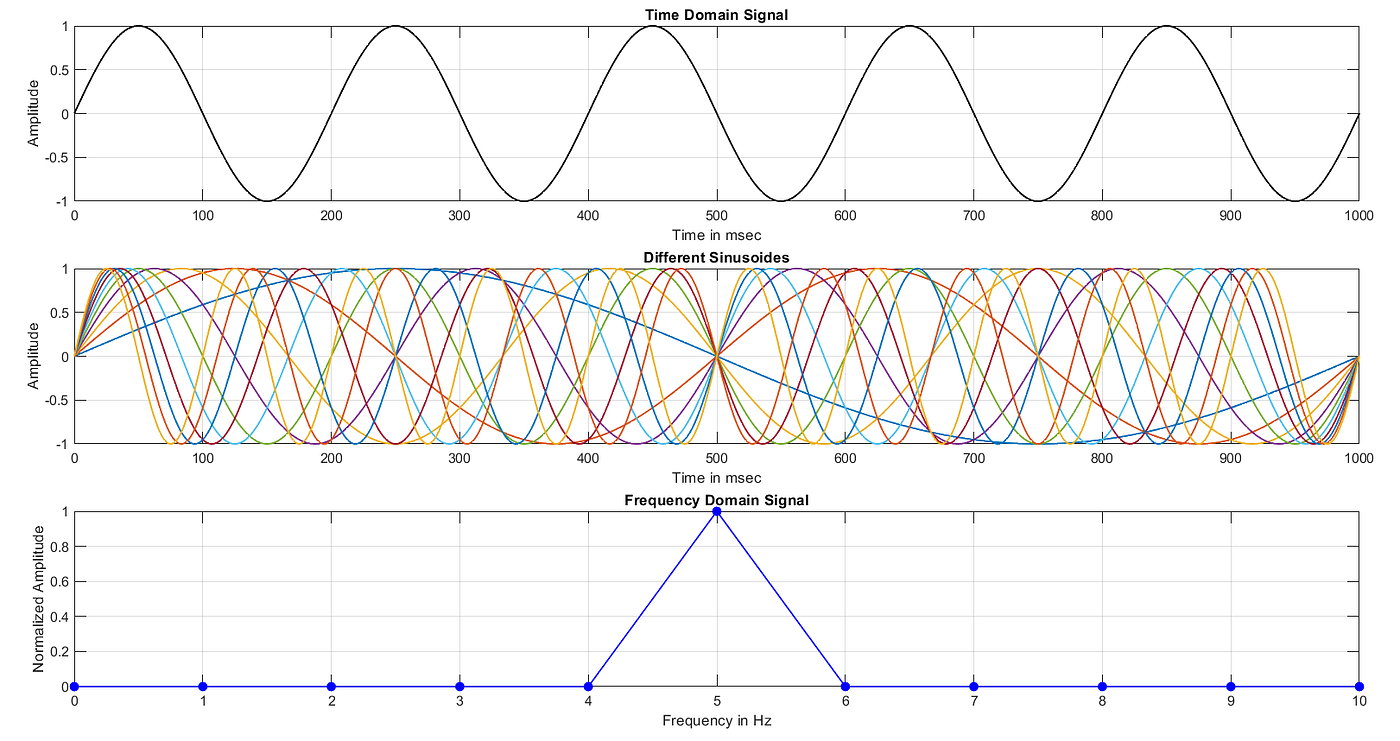Figure 5. Concept of Fourier TransformEq3. The Euler’s Formula
1. By fundamental limit of uncertainty, it can not provide simultaneous time and frequency localization. To know a highly certain spectrum of a signal, a long-duration time-domain signal is required. Hence it is not suitable for non-stationary signals.
2. Fourier transform can not completely resolve information when periodic oscillations are superimposed on decaying signals.

--

--